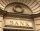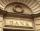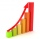# Two years

Roy deposited 50,000.00 into his account paying 4% annual interest compounded semi annually. How much is the interest after 2 years?

i =  4121.608

### Step-by-step explanation:Did you find an error or inaccuracy? Feel free to write us. Thank you!Tips to related online calculators
Do you want to convert time units like minutes to seconds?

## Related math problems and questions:

• Annual interestA loan of 10 000 euro is to be repaid in annual payments over 10 years. Assuming a fixed 10% annual interest rate compounded annually, calculate: (a) the amount of each annual repayment (b) the total interest paid.
• Future value investmentPoseidon deposited 2,747 golden drachmas in a Mount Olympus college savings account to ensure Percy can go to college. It pays 0.04 (percent in decimal form) annual interest. After 11 years, he withdraws the money. How much more money would he have if the
• If you 2If you deposit $4000 into an account paying 9% annual interest compounded monthly, how long until there is$10000 in the account?
• You takeYou take out Php 20 000 loan at 5% interest rate. If the interest is compounded annually, a. Give an exponential model for the situation b. How much Will you owe after 10 years?
• How much 2How much money would you need to deposit today at 5% annual interest compounded monthly to have $2000 in the account after 9 years? • If you 4If you deposit$2500 in an account paying 11% annual interest compounded quarterly, how long until there is the $4500 in the account? • Suppose 3Suppose that a couple invested Php 50 000 in an account when their child was born, to prepare for the child's college education. If the average interest rate is 4.4% compounded annually, a, Give an exponential model for the situation b, Will the money be • Compound interestCompound interest: Clara deposited CZK 100,000 in the bank with an annual interest rate of 1.5%. Both money and interest remain deposited in the bank. How many CZK will be in the bank after 3 years? • If you 3If you deposit$4500 at 5% annual interest compound quarterly, how much money will be in the account after 10 years?
• Compound InterestIf you deposit $6000 in an account paying 6.5% annual interest compounded quarterly, how long until there is$12600 in the account?
• Compound interest 3After 8 years, what is the total amount of a compound interest investment of $25,000 at 3% interest, compounded quarterly? (interest is now dream - in the year 2019) • Combined interestCombined interest: Carol has deposited CZK 100,000 in the bank with an annual interest rate of 1.5%. The money was put into the account 5.5.2016 and withdraw them all before Christmas 20.12.2016. How much money did she withdraw? • Semiannually compound interestIf you deposit$5000 into an account paying 8.25% annual interest compounded semiannually, how long until there is $9350 in the account? • Compound interest 43600 dollars is placed in an account with an annual interest rate of 9%. How much will be in the account after 25 years, to the nearest cent? • DepositOh I total of$15,000 deposited into simple interest accounts the annual simple interest rate on one account at 6% the annual simple interest rate on the second account at 7% how much should be invested in each account so that the total interest earned is
• SavingsThe depositor regularly wants to invest the same amount of money in the financial institution at the beginning of the year and wants to save 10,000 euros at the end of the tenth year. What amount should he deposit if the annual interest rate for the annua
• 3 years savingsSimple interest problem. Tereza has deposited CZK 100,000 in the bank with an annual interest rate of 1.5%. Interest is added each year and left aside. How many CZK (crowns) will have total after 3 years?